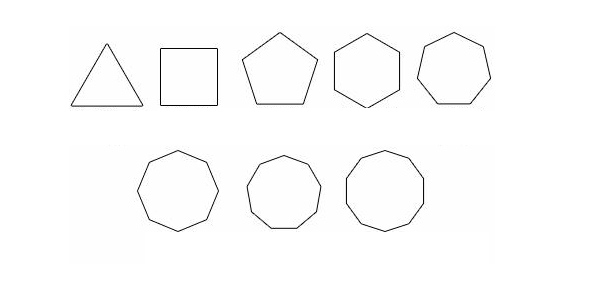# Angles Of Polygons 2

2 Questions | Total Attempts: 77SettingsSum of ext angles = 360sum of int angles = (n-2)180polygon with n sides has n angles.

Related Topics
• 1.
• A.

9

• B.

10

• C.

11

• D.

12

• E.

13

• 2.
Name the regular polygon whose interior angle is four times as large as its exterior angle.
• A.

Square

• B.

Regular hexagon

• C.

Regular octagon

• D.

Regular decagon

• E.

Regular nonagon